# B. A Summary of One-Dimensional Radiative-Convective Equilibrium Modeling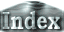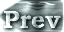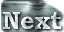### c. Dependence of the Upper Limit of Radiation on Relative Humidity

The cases considered so far are those for a saturated troposphere. In light of application to 3D calculations, cases for an unsaturated troposphere also need to be discussed.

#### The Komabayashi-Ingersoll Limit with Considering Relative Humidity

 The value of specific humidity is constant in the stratosphere. If the tropopause is unsaturated, the relationships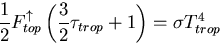and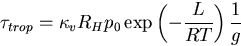hold. Here, RH represents relative humidity. Rearranging these equations for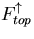top leads to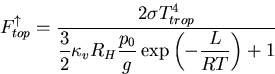Figure 1 shows the maximum value ofevaluated from this equation. As illustrated in the figure,increases rapidly with decreasing relative humidity. For example,is approximately 700 W/m2 for Rh = 0.1.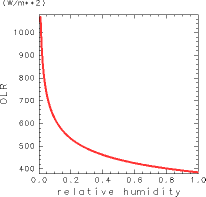Figure 1: The upper limit of OLR taking relative humidity at the tropopause into consideration.

#### The Maximum value of OLR based on the Solution of the Radiative-Convective Equilibrium Solution

 The subsequent discussion examines changes of the maximum value of OLR obtained by the 1D radiative-convective equilibrium model with considering relative humidity of troposphere. For this examination, radiation calculations are performed to obtain equilibrium solutions by first constructing an adiabatic line and then reducing the water vapor by the exact amount of the value of specified relative humidity. Figure 2 shows the surface temperature-OLR relationship that is obtained by setting relative humidity to various values. Both the maximum value of OLR and the asymptotic values of OLR at high temperature limits become large with decreasing relative humidity. When relative humidity is 60 %, the maximum value of OLR and the asymptotic value of OLR at high temperature limits are 390 W/m2 and 320 W/m2, respectively.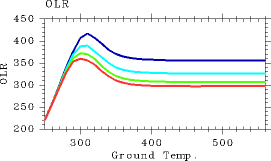Figure 2: The relationships between surface temperature (K) and OLR (W/m2) obtained by the 1D radiative-convective equilibrium model by varying relative humidity. Red: relative humidity of 100 % (identical to Nakajima et al., 1992). Green: relative humidity of 80 %. Aqua: relative humidity of 60 %. Blue: relative humidity of 40 %.

 B.c. Dependence of the Upper Limit of Radiation on Relative Humidity